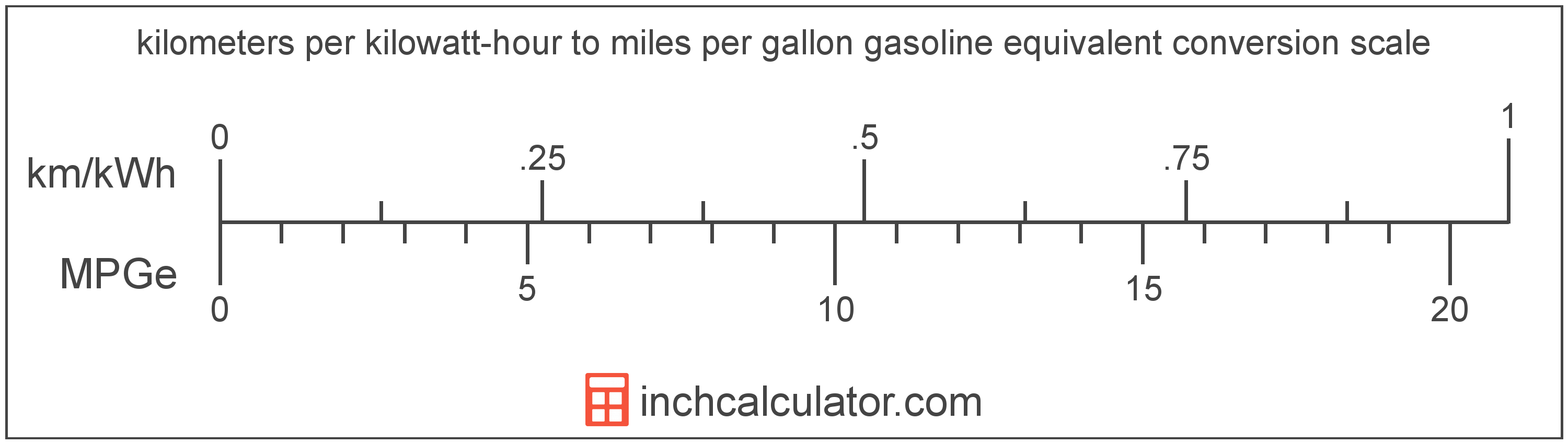# Miles per Gallon Gasoline Equivalent to Kilometers per Kilowatt-Hour Converter (MPGe to Km/kWh)

Enter the electric car efficiency in miles per gallon gasoline equivalent below to get the value converted to kilometers per kilowatt-hour.

Results in Kilometers per Kilowatt-Hour:1 MPGe = 0.047748 km/kWh

Do you want to convert km/kWh to MPGe?

## How to Convert Miles per Gallon Gasoline Equivalent to Kilometers per Kilowatt-Hour

Convert miles per gallon gasoline equivalent to kilometers per kilowatt-hour with this simple formula:

km/kWh = MPGe × 160.9344 ÷ 3370.5

Insert the MPGe efficiency measurement in the formula and then solve to find the result.

For example, let's convert 50 MPGe to km/kWh:

50 MPGe = ( 50 × 160.9344 ÷ 3370.5 ) = 2.387397 km/kWhMiles per gallon gasoline equivalent and kilometers per kilowatt-hour are both units used to measure electric car efficiency. Keep reading to learn more about each unit of measure.

## What Are Miles per Gallon Gasoline Equivalent?

Miles per gallon gasoline equivalent is a way of measuring the energy needed equivalent to a gallon of gasoline to travel a distance in miles.

Because electric vehicles do not consume gasoline, the EPA set an equivalent amount of energy in kilowatt-hours that is equal to one gallon of gasoline. According to the EPA, one gallon of gasoline is equal to 33.705 kilowatt-hours.

The mile per gallon gasoline equivalent is a US customary unit of electric car efficiency. Miles per gallon gasoline equivalent can be abbreviated as MPGe; for example, 1 mile per gallon gasoline equivalent can be written as 1 MPGe.

## What Are Kilometers per Kilowatt-Hour?

Kilometers per kilowatt-hour is a measure of electric vehicle energy efficiency equivalent to the number of kilometers a vehicle can travel using one kilowatt-hour of energy.

The kilometer per kilowatt-hour is an SI unit of electric car efficiency in the metric system. Kilometers per kilowatt-hour can be abbreviated as km/kWh; for example, 1 kilometer per kilowatt-hour can be written as 1 km/kWh.

In the expressions of units, the slash, or solidus (/), is used to express a change in one or more units relative to a change in one or more other units.

## Mile per Gallon Gasoline Equivalent to Kilometer per Kilowatt-Hour Conversion Table

Table showing various mile per gallon gasoline equivalent measurements converted to kilometers per kilowatt-hour.
Miles Per Gallon Gasoline Equivalent Kilometers Per Kilowatt-hour
1 MPGe 0.047748 km/kWh
2 MPGe 0.095496 km/kWh
3 MPGe 0.143244 km/kWh
4 MPGe 0.190992 km/kWh
5 MPGe 0.23874 km/kWh
6 MPGe 0.286488 km/kWh
7 MPGe 0.334236 km/kWh
8 MPGe 0.381983 km/kWh
9 MPGe 0.429731 km/kWh
10 MPGe 0.477479 km/kWh
11 MPGe 0.525227 km/kWh
12 MPGe 0.572975 km/kWh
13 MPGe 0.620723 km/kWh
14 MPGe 0.668471 km/kWh
15 MPGe 0.716219 km/kWh
16 MPGe 0.763967 km/kWh
17 MPGe 0.811715 km/kWh
18 MPGe 0.859463 km/kWh
19 MPGe 0.907211 km/kWh
20 MPGe 0.954959 km/kWh
21 MPGe 1.0027 km/kWh
22 MPGe 1.0505 km/kWh
23 MPGe 1.0982 km/kWh
24 MPGe 1.146 km/kWh
25 MPGe 1.1937 km/kWh
26 MPGe 1.2414 km/kWh
27 MPGe 1.2892 km/kWh
28 MPGe 1.3369 km/kWh
29 MPGe 1.3847 km/kWh
30 MPGe 1.4324 km/kWh
31 MPGe 1.4802 km/kWh
32 MPGe 1.5279 km/kWh
33 MPGe 1.5757 km/kWh
34 MPGe 1.6234 km/kWh
35 MPGe 1.6712 km/kWh
36 MPGe 1.7189 km/kWh
37 MPGe 1.7667 km/kWh
38 MPGe 1.8144 km/kWh
39 MPGe 1.8622 km/kWh
40 MPGe 1.9099 km/kWh

## References

1. United States Environmental Protection Agency, Technology, https://www3.epa.gov/otaq/gvg/learn-more-technology.htm